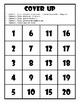# Math Facts to 20 Game Game Cover Up Math Game Math Facts to 20 CentersSubject
Resource Type
File Type

PDF

(618 KB|6 pages)
Standards
• Product Description
• StandardsNEW

Two options included - one to practice facts through 20 and the other with facts through 12 to allow for differentiation. In this math game students roll two dice (or roll one twice) or use a number spinner twice and find the sum to get a number - example if you roll a 2 and 6 your number is 8. With their number students then see how many numbers they can cover up! This game is great for practicing both addition and subtraction facts since they can cover up the numbers in a variety of ways. Such as cover the sum, cover two numbers that create the same sum, or cover two numbers that you can subtract and find a difference that’s the same number.

Example: If the sum of your dice roll is 8 - Option one cover 8. Option two cover 2 and 6, or 5 and 3 (5+3=8) etc. anything that equals 8. Option three cover 12 and 4 since 12-4=8.

There are also several variations on the game with playing alone or with a partner, 4 in a row, cover a column, cover the whole game board etc.

My students love this game!

*This game is also really great if you purchase 10-sided polyhedral dice (numbers 0-9) for a wider array of numbers!

Fluently add and subtract within 20 using mental strategies. By end of Grade 2, know from memory all sums of two one-digit numbers.
Add and subtract within 20, demonstrating fluency for addition and subtraction within 10. Use strategies such as counting on; making ten (e.g., 8 + 6 = 8 + 2 + 4 = 10 + 4 = 14); decomposing a number leading to a ten (e.g., 13 - 4 = 13 - 3 - 1 = 10 - 1 = 9); using the relationship between addition and subtraction (e.g., knowing that 8 + 4 = 12, one knows 12 - 8 = 4); and creating equivalent but easier or known sums (e.g., adding 6 + 7 by creating the known equivalent 6 + 6 + 1 = 12 + 1 = 13).
Add within 100, including adding a two-digit number and a one-digit number, and adding a two-digit number and a multiple of 10, using concrete models or drawings and strategies based on place value, properties of operations, and/or the relationship between addition and subtraction; relate the strategy to a written method and explain the reasoning used. Understand that in adding two-digit numbers, one adds tens and tens, ones and ones; and sometimes it is necessary to compose a ten.
Total Pages
6 pages
N/A
Teaching Duration
N/A
Report this Resource to TpT
Reported resources will be reviewed by our team. Report this resource to let us know if this resource violates TpT’s content guidelines.